Related Articles

# IPL Score Prediction using Deep Learning

• Difficulty Level : Medium
• Last Updated : 04 Jul, 2021

Since the dawn of the IPL in 2008, it has attracted viewers all around the globe. A high level of uncertainty and last moment nail biters has urged fans to watch the matches. Within a short period, IPL has become the highest revenue-generating league of cricket. In a cricket match, we often see the scoreline showing the probability of the team winning based on the current match situation. This prediction is usually done with the help of Data Analytics. Before when there were no advancements in machine learning, the prediction was usually based on intuitions or some basic algorithms. The above picture clearly tells you how bad is taking run rate as a single factor to predict the final score in a limited-overs cricket match.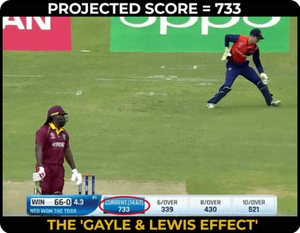Attention reader! Don’t stop learning now. Get hold of all the important Machine Learning Concepts with the Machine Learning Foundation Course at a student-friendly price and become industry ready.

Being a cricket fan, visualizing the statistics of cricket is mesmerizing. We went through various blogs and found out patterns that could be used for predicting the score of IPL matches beforehand.

### Why Deep Learning?

We humans can’t easily identify patterns from huge data and thus here, machine learning and deep learning comes into play. It learns how the players and teams have performed against the opposite team previously and trains the model accordingly. Using only machine learning algorithm gives a moderate accuracy therefore we used deep learning which gives much better performance than our previous model and considers the attributes which can give accurate results.

### Tools used:

• Jupyter Notebook / Google colab
• Visual Studio

### Technology used:

• Machine Learning.
• Deep Learning
• Well, for the smooth running of the project we’ve used few libraries like NumPy, Pandas, Scikit-learn, TensorFlow, and Matplotlib.

### The architecture of model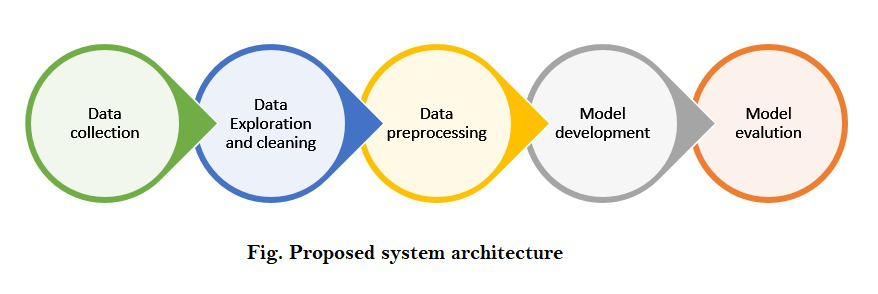### Step-by-step implementation:

First, let’s import all the necessary libraries:

## Python3

 `import` `pandas as pd``import` `numpy as np``import` `matplotlib.pyplot as plt``import` `seaborn as sns``from` `sklearn ``import` `preprocessing`

Step 1: Understanding the dataset!

When dealing with cricket data, Cricsheet is considered as an appropriate platform for gathering the data and thus we took the data from https://cricsheet.org/downloads/ipl.zip. It contains data from the year 2007 to 2021. For better accuracy of our model, we used IPL players’ stats to analyze their performance from here. This dataset contains details of every IPL player from the year 2016 – 2019.

Step 2: Data cleaning and formatting

We imported both the datasets using .read_csv() method into a dataframe using pandas and displayed the first 5 rows of each dataset. We did some changes to our dataset like added a new column named “y” which had the runs scored in the first 6 overs from that particular inning.

## Python3

 `ipl ``=` `pd.read_csv(``'ipl_dataset.csv'``)``ipl.head()`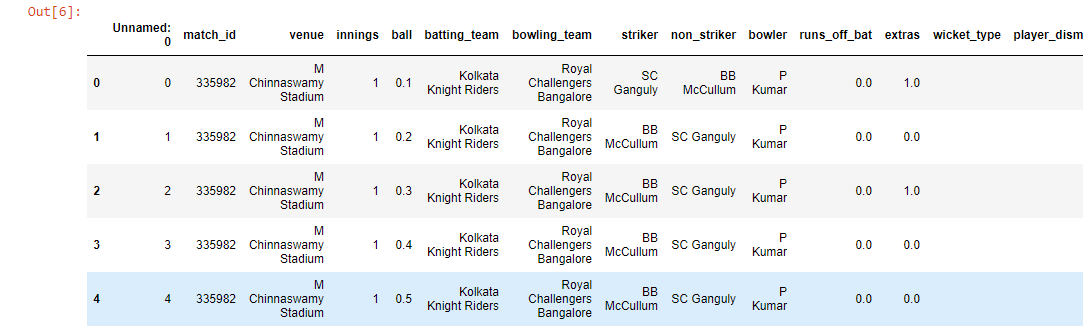## Python3

 `data ``=` `pd.read_csv(``'IPL Player Stats - 2016 till 2019.csv'``)``data.head()`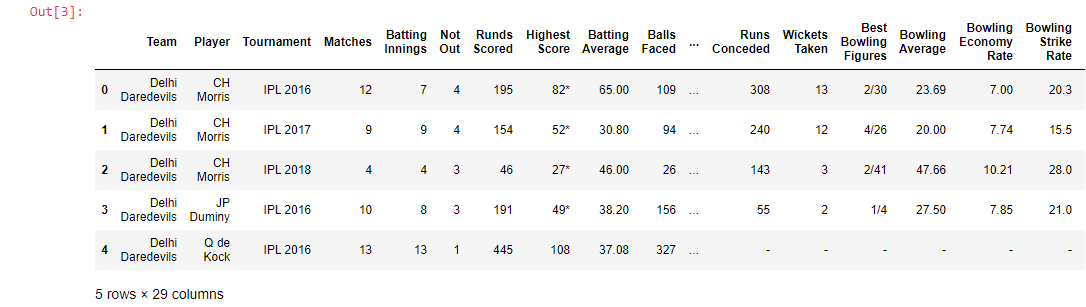Now, we will merge both datasets.

## Python3

 `ipl``=` `ipl.drop([``'Unnamed: 0'``,``'extras'``,``'match_id'``, ``'runs_off_bat'``],axis ``=` `1``)``new_ipl ``=` `pd.merge(ipl,data,left_on``=``'striker'``,right_on``=``'Player'``,how``=``'left'``)``new_ipl.drop([``'wicket_type'``, ``'player_dismissed'``],axis``=``1``,inplace``=``True``)``new_ipl.columns`

After merging the columns and removing new unwanted columns, we have the following columns left. Here’s the modified dataset.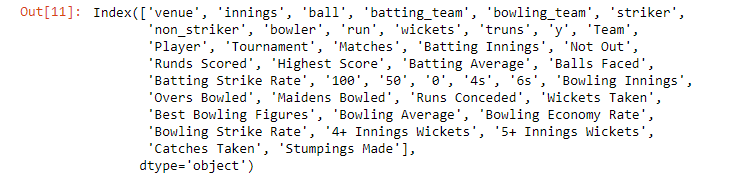There are various ways to fill null values in our dataset. Here I am simply replacing the categorical values which are nan with ‘.’

## Python3

 `str_cols ``=` `new_ipl.columns[new_ipl.dtypes``=``=``object``]``new_ipl[str_cols] ``=` `new_ipl[str_cols].fillna(``'.'``)`

Step 3: Encoding the categorical data to numerical values.

For the columns to be able to assist the model in the prediction, the values should make some sense to the computers. Since they (still) don’t have the ability to understand and draw inferences from the text, we need to encode the strings to numeric categorical values. While we may choose to do the process manually, the Scikit-learn library gives us an option to use LabelEncoder.

## Python3

 `listf ``=` `[]`` ` `for` `c ``in` `new_ipl.columns:``    ``if` `new_ipl.dtype``=``=``object``:``        ``print``(c,``"->"` `,new_ipl.dtype)``        ``listf.append(c)`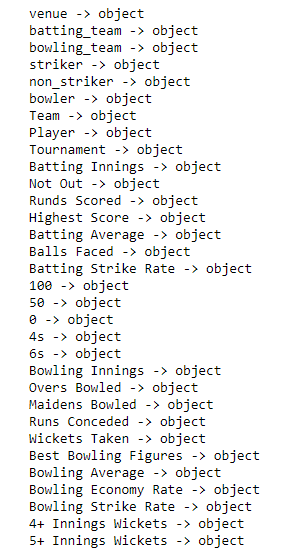## Python3

 `a1 ``=` `new_ipl[``'venue'``].unique()``a2 ``=` `new_ipl[``'batting_team'``].unique()``a3 ``=` `new_ipl[``'bowling_team'``].unique()``a4 ``=` `new_ipl[``'striker'``].unique()``a5 ``=` `new_ipl[``'bowler'``].unique()`` ` `def` `labelEncoding(data):``    ``dataset ``=` `pd.DataFrame(new_ipl)``    ``feature_dict ``=``{}``     ` `    ``for` `feature ``in` `dataset:``        ``if` `dataset[feature].dtype``=``=``object``:``            ``le ``=` `preprocessing.LabelEncoder()``            ``fs ``=` `dataset[feature].unique()``            ``le.fit(fs)``            ``dataset[feature] ``=` `le.transform(dataset[feature])``            ``feature_dict[feature] ``=` `le``             ` `    ``return` `dataset`` ` `labelEncoding(new_ipl)`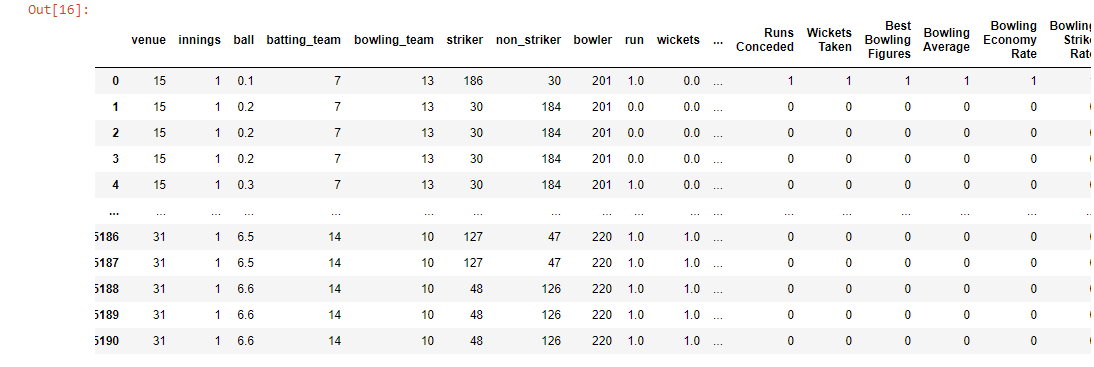## Python3

 `ip_dataset ``=` `new_ipl[[``'venue'``,``'innings'``, ``'batting_team'``, ``                      ``'bowling_team'``, ``'striker'``, ``'non_striker'``,``                      ``'bowler'``]]`` ` `b1 ``=` `ip_dataset[``'venue'``].unique()``b2 ``=` `ip_dataset[``'batting_team'``].unique()``b3 ``=` `ip_dataset[``'bowling_team'``].unique()``b4 ``=` `ip_dataset[``'striker'``].unique()``b5 ``=` `ip_dataset[``'bowler'``].unique()``new_ipl.fillna(``0``,inplace``=``True``)`` ` `features``=``{}`` ` `for` `i ``in` `range``(``len``(a1)):``    ``features[a1[i]]``=``b1[i]``for` `i ``in` `range``(``len``(a2)):``    ``features[a2[i]]``=``b2[i]``for` `i ``in` `range``(``len``(a3)):``    ``features[a3[i]]``=``b3[i]``for` `i ``in` `range``(``len``(a4)):``    ``features[a4[i]]``=``b4[i]``for` `i ``in` `range``(``len``(a5)):``    ``features[a5[i]]``=``b5[i]``     ` `features`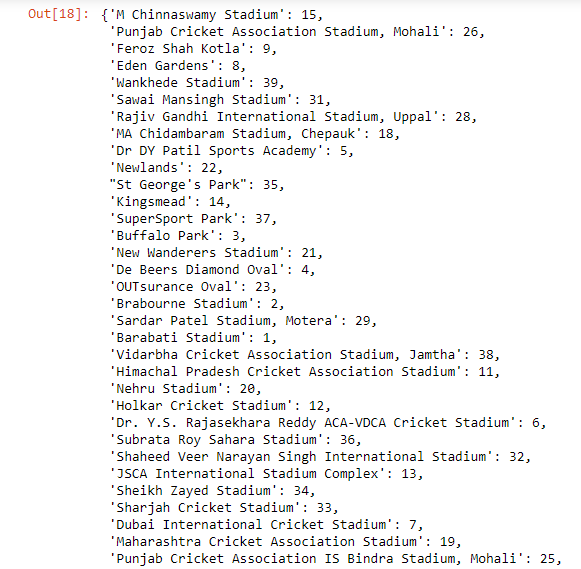Step 4: Feature Engineering and Selection

Our dataset contains multiple columns, but we can’t take these many inputs from users thus we have taken the selected amount of features as input and divided them into X and y. We will then divide our data into train sets and test set before using a machine learning algorithm.

## Python3

 `X ``=` `new_ipl[[``'venue'``, ``'innings'``,``'batting_team'``,``             ``'bowling_team'``, ``'striker'``,``'bowler'``]].values``y ``=` `new_ipl[``'y'``].values`` ` `from` `sklearn.model_selection ``import` `train_test_split`` ` `X_train, X_test, y_train, y_test ``=` `train_test_split(``  ``X, y, test_size``=``0.33``, random_state``=``42``)`

Comparing these large numerical values by our model will be difficult so it is always a better choice to scale your data before processing it. Here we are using MinMaxScaler from sklearn.preprocessing which is recommended when dealing with deep learning.

## Python3

 `from` `sklearn.preprocessing ``import` `MinMaxScaler``scaler ``=` `MinMaxScaler()`` ` `X_train ``=` `scaler.fit_transform(X_train)``X_test ``=` `scaler.transform(X_test)`

Note: We cannot fit X_test as it is the data which is to be predicted.

Step 5: Building, Training & Testing the Model

Here comes the most exciting part of our project, Building our model! Firstly, we will import Sequential from tensorflow.keras.models Also, we will import Dense & Dropout from tensorflow.keras.layers as we will be using multiple layers.

## Python3

 `from` `tensorflow.keras.models ``import` `Sequential``from` `tensorflow.keras.layers ``import` `Dense,Dropout``from` `tensorflow.keras.callbacks ``import` `EarlyStopping`

EarlyStopping is used to avoid overfitting. What early stopping basically does is, it stops calculating the losses when ‘val_loss’ increases than ‘loss’. Val_loss curve should always be below val curve. When it is found that the difference between ‘val_loss’ and ‘loss’ is becomes constant, it stops training.

## Python3

 `model ``=` `Sequential()`` ` `model.add(Dense(``43``, activation``=``'relu'``))``model.add(Dropout(``0.5``))`` ` `model.add(Dense(``22``, activation``=``'relu'``))``model.add(Dropout(``0.5``))`` ` `model.add(Dense(``11``, activation``=``'relu'``))``model.add(Dropout(``0.5``))`` ` `model.add(Dense(``1``))`` ` `model.``compile``(optimizer``=``'adam'``, loss``=``'mse'``)`

Here, we have created 2 hidden layers and reduced the number of neurons as we want the final output to be 1. Then while compiling our model we used adam optimizer and loss as mean squared error.  Now, let’s start training our model with epochs=400.

## Python3

 `model.fit(x``=``X_train, y``=``y_train, epochs``=``400``, ``          ``validation_data``=``(X_test,y_test),``          ``callbacks``=``[early_stop] )`

It will take some time because of a huge number of samples and epochs and will output the ‘loss’ and ‘val_loss’ of each sample as below.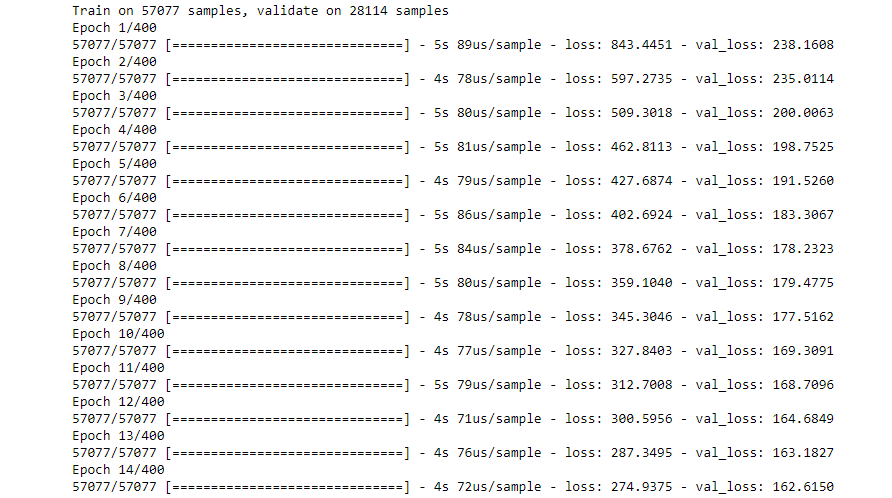After the training is complete, let us visualize our model’s losses.

## Python3

 `model_losses ``=` `pd.DataFrame(model.history.history)``model_losses.plot()`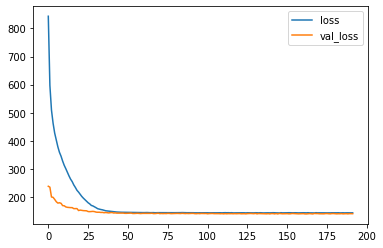As we can see our model is having absolutely perfect behavior!

Step 6: Predictions!

Here we come to the final part of our project where we will be predicting our X_test. Then we will create a dataframe that would show us the actual values and the predicted values.

## Python3

 `predictions ``=` `model.predict(X_test)``sample ``=` `pd.DataFrame(predictions,columns``=``[``'Predict'``])``sample[``'Actual'``]``=``y_test``sample.head(``10``)`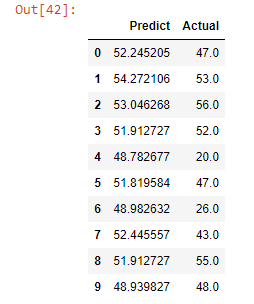As we can see, our model is predicting quite well. It is giving us almost similar scores. To find out more accurately the difference between actual and predicted scores, performance metrics will show us the error rate using mean_absolute_error and mean_squared_error from sklearn.metrics

Have a look at our front-end: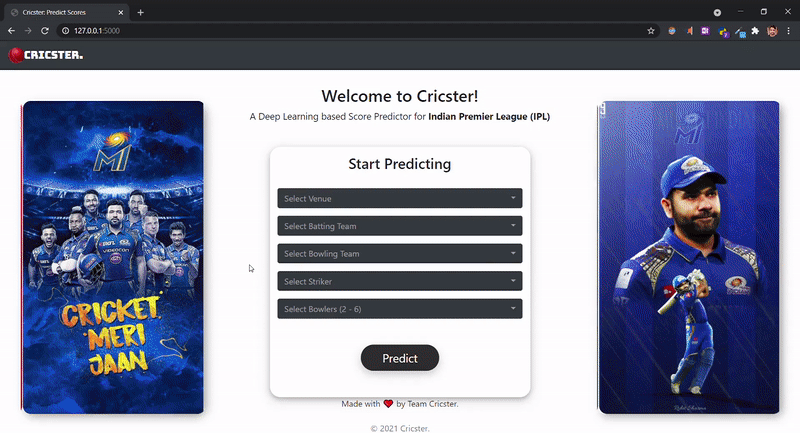cricster.com

Performance Metrics!

## Python3

 `from` `sklearn.metrics ``import` `mean_absolute_error,mean_squared_error`` ` `mean_absolute_error(y_test,predictions)`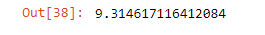## Python3

 `np.sqrt(mean_squared_error(y_test,predictions))`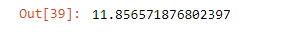Let’s take a look at our model! 🙂

### Team Member:

• Shravani Rajguru
• Hrushabh Kale